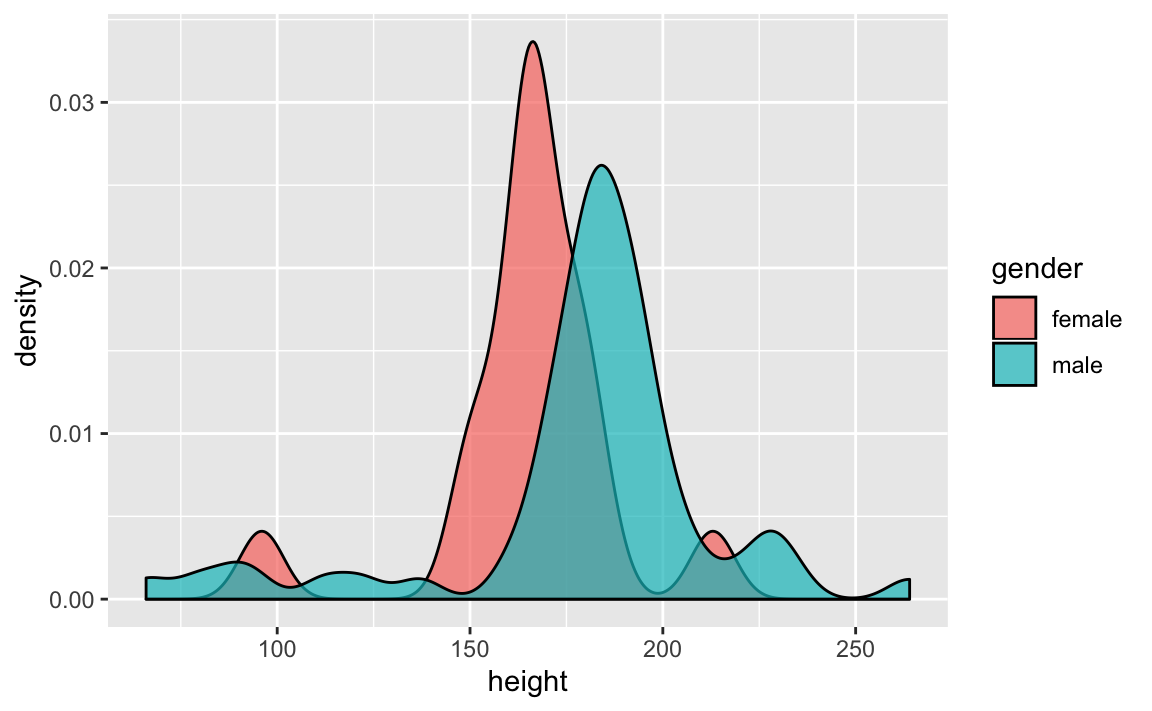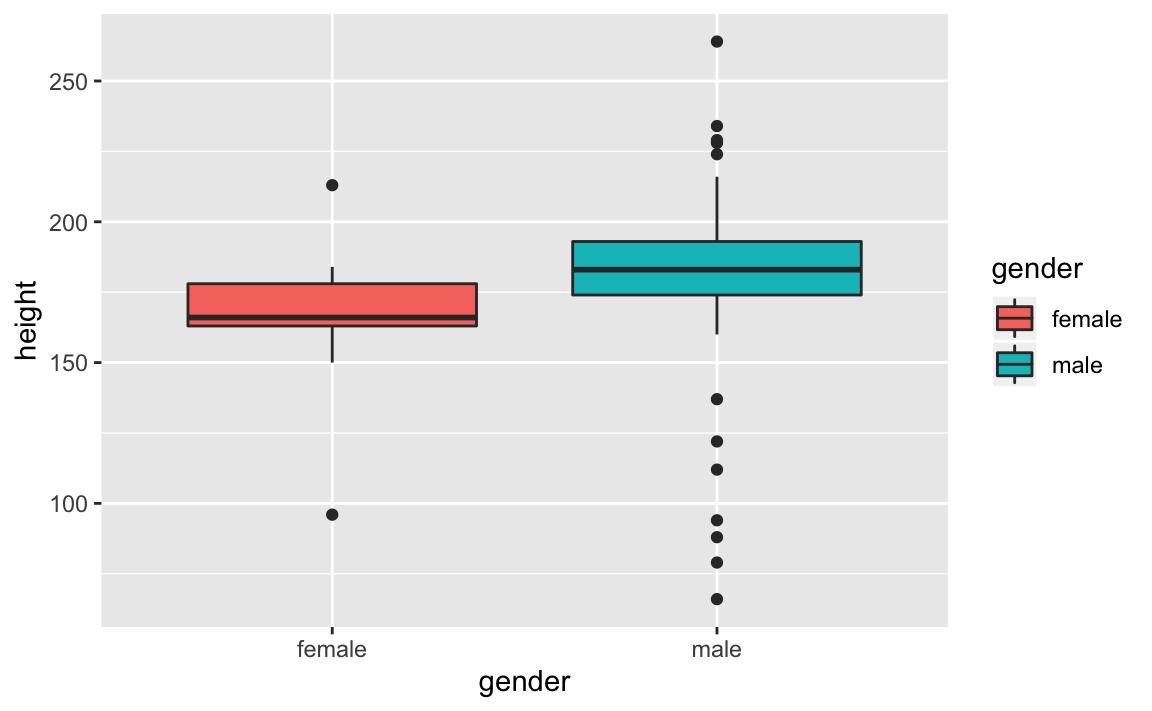23 Pipes and More dplyr

23.1 Introduction

In previous chapters, we started to manipulate data tables (e.g. data.frame, tibble) with functions provided by the R package "dplyr".

Having been exposed to the dplyr paradigm, let’s compare R base manipulation against the various dplyr syntax flavors.

23.1.1 Starwars Data Set

In this tutorial we are going to use the data set starwars that comes in "dplyr":

# data set
starwars
#> # A tibble: 87 x 13
#>    name  height  mass hair_color skin_color eye_color birth_year gender
#>    <chr>  <int> <dbl> <chr>      <chr>      <chr>          <dbl> <chr>
#>  1 Luke…    172    77 blond      fair       blue            19   male
#>  2 C-3PO    167    75 <NA>       gold       yellow         112   <NA>
#>  3 R2-D2     96    32 <NA>       white, bl… red             33   <NA>
#>  4 Dart…    202   136 none       white      yellow          41.9 male
#>  5 Leia…    150    49 brown      light      brown           19   female
#>  6 Owen…    178   120 brown, gr… light      blue            52   male
#>  7 Beru…    165    75 brown      light      blue            47   female
#>  8 R5-D4     97    32 <NA>       white, red red             NA   <NA>
#>  9 Bigg…    183    84 black      light      brown           24   male
#> 10 Obi-…    182    77 auburn, w… fair       blue-gray       57   male
#> # … with 77 more rows, and 5 more variables: homeworld <chr>, species <chr>,
#> #   films <list>, vehicles <list>, starships <list>

For illustration purposes, let’s consider a relatively simple example. Say we are interested in calculating the average (mean) height for both female and male individuals. Let’s discuss how to find the solution under the base R approach, as well as the dplyr approach.

23.2 Base R approach

Let’s see how to use base R operations to find the average height of individuals with gender female and male.

# identify female and male individuals
# (comparison operations)
which_females <- starwars$gender == 'female' which_males <- starwars$gender == 'male'
# select the height values of females and males
# (via logical subsetting)
height_females <- starwars$height[which_females] height_males <- starwars$height[which_males]
# calculate averages (removing missing values)
avg_ht_female <- mean(height_females, na.rm = TRUE)
avg_ht_male <- mean(height_males, na.rm = TRUE)
# optional: display averages in a vector
c('female' = avg_ht_female, 'male' = avg_ht_male)
#> female   male
#>    165    179

All the previous code can be written with more compact expressions:

# all calculations in a couple of lines of code
c("female" = mean(starwars$height[starwars$gender == 'female'], na.rm = TRUE),
"male" = mean(starwars$height[starwars$gender == 'male'], na.rm = TRUE)
)
#> female   male
#>    165    179

23.3 With "dplyr"

The behavior of "dplyr" is functional in the sense that function calls don’t have side-effects. You must always save their results in order to keep them in an object (in memory). This doesn’t lead to particularly elegant code, especially if you want to do many operations at once.

23.3.1 Option 1) Step-by-step

You either have to do it step-by-step:

# manipulation step-by-step
gender_height <- select(starwars, gender, height)
fem_male_height <- filter(gender_height,
gender == 'female' | gender == 'male')
height_by_gender <- group_by(fem_male_height, gender)
summarise(height_by_gender, mean(height, na.rm = TRUE))
#> # A tibble: 2 x 2
#>   gender mean(height, na.rm = TRUE)
#>   <chr>                         <dbl>
#> 1 female                         165.
#> 2 male                           179.

23.3.2 Option 2) Nested (embedded) code

Or if you don’t want to name the intermediate results, you need to wrap the function calls inside each other:

summarise(
group_by(
filter(select(starwars, gender, height),
gender == 'female' | gender  == 'male'),
gender),
mean(height, na.rm = TRUE)
)
#> # A tibble: 2 x 2
#>   gender mean(height, na.rm = TRUE)
#>   <chr>                         <dbl>
#> 1 female                         165.
#> 2 male                           179.

This is difficult to read because the order of the operations is from inside to out. Thus, the arguments are a long way away from the function.

23.3.3 Option 3) Piping

To get around the problem of nesting functions, "dplyr" also provides the %>% operator from the R package "magrittr".

What does the piper %>% do? Here’s a conceptual example:

x %>% f(y)

x %>% f(y) turns into f(x, y) so you can use it to rewrite multiple operations that you can read left-to-right, top-to-bottom.

Here’s how to use the piper to calculate the average height for female and male individuals:

avg_height_by_gender <- starwars %>%
select(gender, height) %>%
filter(gender == 'female' | gender == 'male') %>%
group_by(gender) %>%
summarise(avg = mean(height, na.rm = TRUE))
avg_height_by_gender
#> # A tibble: 2 x 2
#>   gender   avg
#>   <chr>  <dbl>
#> 1 female  165.
#> 2 male    179.
avg_height_by_gender$avg #>  165 179 Here’s another example in which we calculate the mean height and mean mass of species Droid, Ewok, and Human; arranging the rows of the tibble by mean height, in descending order: starwars %>% select(species, height, mass) %>% filter(species %in% c('Droid', 'Ewok', 'Human')) %>% group_by(species) %>% summarise( mean_height = mean(height, na.rm = TRUE), mean_mass = mean(mass, na.rm = TRUE) ) %>% arrange(desc(mean_height)) #> # A tibble: 3 x 3 #> species mean_height mean_mass #> <chr> <dbl> <dbl> #> 1 Human 177. 82.8 #> 2 Droid 140 69.8 #> 3 Ewok 88 20 23.4 Pipes and Plots You can also the %>% operator to chain dplyr commands with ggplot commans (and other R commands). The following examples combine some data manipulation to filter() female and males individuals, in order to graph a density plot of height starwars %>% filter(gender %in% c('female', 'male')) %>% ggplot(aes(x = height, fill = gender)) + geom_density(alpha = 0.7) #> Warning: Removed 5 rows containing non-finite values (stat_density).Here’s another example in which instead of graphing density plots, we graph boxplots of height for female and male individuals: starwars %>% filter(gender %in% c('female', 'male')) %>% ggplot(aes(x = gender, y = height, fill = gender)) + geom_boxplot() #> Warning: Removed 5 rows containing non-finite values (stat_boxplot).23.5 More Pipes Often, you will work with functions that don’t take data frames (or tibbles) as inputs. A typical example is the base plot() function used to produce a scatterplot; you need to pass vectors to plot(), not data frames. In this situations you might find the %$% operator extremely useful.

library(magrittr)

The %$% operator, also from the package "magrittr", is a cousin of the %>% operator. What %$% does is to extract variables in a data frame so that you can refer to them explicitly. Let’s see a quick example:

starwars %>%
filter(gender %in% c('female', 'male')) %$% plot(x = height, y = mass, col = factor(gender), las = 1) 23.6 Exercises Consider the following data frame dat first last gender title gpa 1 Jon Snow male lord 3 2 Arya Stark female princess 3 3 Tyrion Lannister male master 4 4 Daenerys Targaryen female khaleesi 3 5 Yara Greyjoy female princess 4 1) What is the output of the following command? dat %>% select(gender, gpa) %>% filter(gender == 'male') %>% summarise(max(gpa)) 2) What is the output of the following command? dat %>% select(first, last) %>% arrange(desc(first)) 3) What is the output of the following command? dat %>% filter(gender == 'female' | title != 'khaleesi') %>% select(title) Consider the following data frame dat Month Week Temp Wind 1 5 1 67 7.4 2 5 2 72 8.0 3 5 3 74 12.6 4 5 4 62 11.5 5 6 1 78 8.6 6 6 2 74 9.7 7 6 3 67 16.1 8 6 4 84 9.2 4) What is the output of the following command? Try to guess the output without running the command. dat %>% filter(Month == 5 & (Temp > 70 | Wind > 10)) 5) What is the output of the following command? Try to guess the output without running the command. dat %>% summarise(max_temp = max(Temp), max_wind = max(Wind)) Consider the following data frame dat first last gender title gpa 1 Jon Snow male lord 3.0 2 Arya Stark female princess 3.5 3 Tyrion Lannister male master 4.0 4 Daenerys Targaryen female khaleesi 3.8 5 Yara Greyjoy female princess 1.5 6) Which of the following commands gives you the following output: #> first #> 1 Yara #> 2 Tyrion #> 3 Jon #> 4 Daenerys #> 5 Arya 1. arrange(select(dat, first), desc(last)) 2. arrange(select(dat, first), first) 3. arrange(select(dat, first), desc(first)) 4. none of the above 7) Which of the following commands gives you the data of female individuals: #> first last gender title gpa #> 1 Arya Stark female princess 3.5 #> 2 Daenerys Targaryen female khaleesi 3.8 #> 3 Yara Greyjoy female princess 1.5 1. filter(dat, gender == female) 2. select(dat, gender == female) 3. group_by(dat, gender == female) 4. none of the above 8) Which of the following commands gives you the following output: #> max(gpa) #> 1 4 1. which.max(dat$gpa)
2. summarise(dat, max(gpa))
3. max(dat\$gpa)
4. none of the above